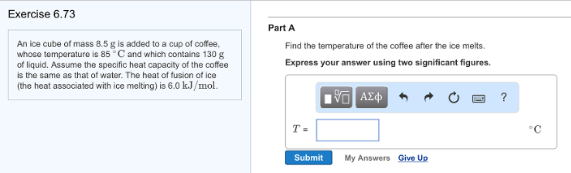# Problem: An ice cube of mass 8.5 g is added to a cup of coffee, whose temperature is 85 °C and which contains 130 g of liquid. Assume the specific heat capacity of the coffee is the same as that of water. The heat of fusion of ice (the heat associated with ice melting) is 6.0 kJ/mol. Part AFind the temperature of the coffee after the ice melts. Express your answer using two significant figures.

###### FREE Expert Solution
95% (105 ratings)###### Problem Details

An ice cube of mass 8.5 g is added to a cup of coffee, whose temperature is 85 °C and which contains 130 g of liquid. Assume the specific heat capacity of the coffee is the same as that of water. The heat of fusion of ice (the heat associated with ice melting) is 6.0 kJ/mol.

Part A

Find the temperature of the coffee after the ice melts. Express your answer using two significant figures.